﻿ 基于降水微物理特征测量仪的雨滴形状观测与分析
 快速检索 中文标题 英文标题 作者中文名 作者英文名 单位中文名 单位英文名 中文关键词 英文关键词 中文摘要 英文摘要 基金项目 全文
 气象2017, Vol. 43Issue (1): 91-100.  DOI: 10.7519/j.issn.1000-0526.2017.01.010### 引用本文 [复制中英文]

[复制中文]
SHU Xiaojian, GAO Taichang, LIU Xichuan, et al, 2017. Observation and Analysis of Raindrop Shape Based on the Precipitation Micro-Physical Characteristics Sensor[J]. Meteorological Monthly, 43(1): 91-100. DOI: 10.7519/j.issn.1000-0526.2017.01.010.
[复制英文]

### 文章历史

2015年12月21日收稿
2016年11月03日收修定稿

1. 解放军理工大学气象海洋学院, 南京 211101
2. 解放军93318部队气象台, 辽宁开原 112300

Observation and Analysis of Raindrop Shape Based on the Precipitation Micro-Physical Characteristics Sensor
SHU Xiaojian1,2, GAO Taichang1, LIU Xichuan1, HU Shuai1, JIANG Shiyang1
1. Institute of Meteorology, PLA University of Science and Technology, Nanjing 211101;
2. Meteorological Observatory, Unit 93318 of PLA, Liaoning, Kaiyuan 112300
Abstract: A self-developed precipitation micro-physical characteristics sensor was applied in field experiment and a large quantity of raindrop images were obtained. The causing reasons and handling methods for outlet raindrop images were discussed, and a vectorized method based on the Akima interpolation under the polar coordinate was proposed in this paper. Then, the characteristics of average raindrop shape and axis ratio distribution were analyzed. The results show that the small drops ( < 1 mm in diameter) have spheroids shapes, the moderate drops ( < 1.0 mm and < 3.0 mm in diameter) have oblate spheroids shapes and the larger drops (>3.0 mm in diameter) have more bulged tops and flattened bases. The mean axis ratio distribution is in good agreement with the empirical relationships, and the deviation between them is smaller than 0.04. The raindrops ranging from 3.4 to 3.8 mm are more spherical than those from empirical model.
Key words: precipitation micro-physical characteristics sensor (PMCS)    Akima interpolation    raindrop shape    axis ratios distribution

Pruppacher and Pitte (1971)通过考虑液体静压力和空气动压力在雨滴表面任意一点的平衡得到PP模型，即直径<2 mm的雨滴基本保持球形，>2 mm的雨滴顶部呈圆滑、底部由平坦状向凹状过渡；Beard and Chuang (1987)在PP模型的基础上考虑雨滴的表面张力，利用拉普拉斯压力平衡方程计算得到BC模型，其大雨滴相对于PP模型底部无凹状；Pruppacher and Klett (1997)指出雨滴形状主要受液体静压力、空气动压力、表面张力、电场力、雨滴内部环流决定；Bhalwankar et al (2015)利用在雨滴风洞实验中添加不同方向和强度的电场，指出随着水平电场强度的增加雨滴平均轴比随之减小；说明雨滴形状在不同的外力影响下具有不同的形状。Thurai and Bringi (2007)Thurai et al (2010)利用2DVD在亚拉巴马(Alabama)的观测资料进行分析，发现在层状云性降水的雨滴形状与Thurai模型相符，但对流性降水的雨滴形状在3.5~3.75 mm较Thurai模型(Thurai et al, 2007)具有更圆的形状，进一步说明在不同的大气环境下雨滴呈现不同的形状；目前，国内外测量雨滴形状以高速线阵CCD扫描为主如2DVD (Kruger and Krajewski, 2002)，但当受水平风影响时，雨滴可能产生水平方向位移，改变雨滴真实形状。

1 PMCS的简介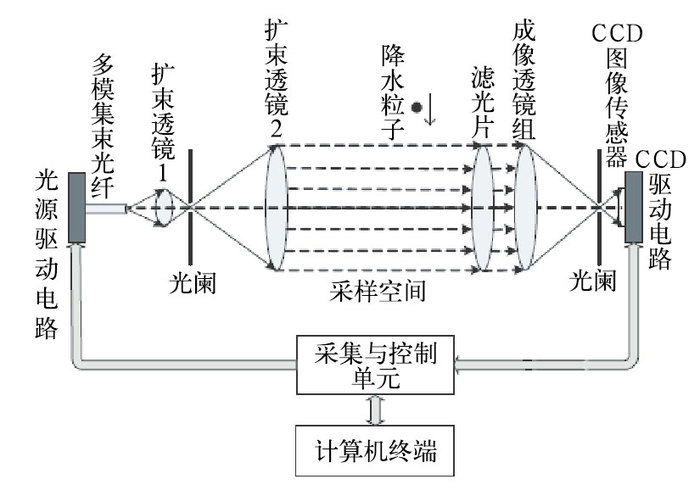图 1 雨滴谱仪的测量原理图 Fig. 1 Schematic diagram of PMCS measure principle

PMCS以平行光作为成像背景场，消除了景深效应对粒子成像的影响。当降水粒子穿过采样空间时，PMCS通过对光源和快门的精密配合实现单帧图像内对该粒子进行两次曝光，然后根据采集的粒子图像得到其大小与形状，并根据粒子先后两次曝光的位置与间隔时间计算水平和垂直速度，详细参数指标见表 1。在PMCS投入实地观测之前，已进行了考虑环境光强与背景光场平行性的小球定标试验(Shu et al, 2015)，确定了基于粒子像元面积的自适应阈值图像处理方法，对捕获的粒子图像依次采用中值滤波、线性空间滤波、腐蚀等图像处理方法进行处理，并根据粒子像元面积与二值化阈值之间函数关系进行二值化，最终可获得较为准确的二值化数值雨滴图像。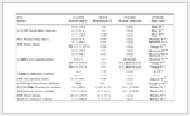表 1 PMCS的主要技术指标 Table 1 Specifications of the PMCS
2 资料获取与异常图像处理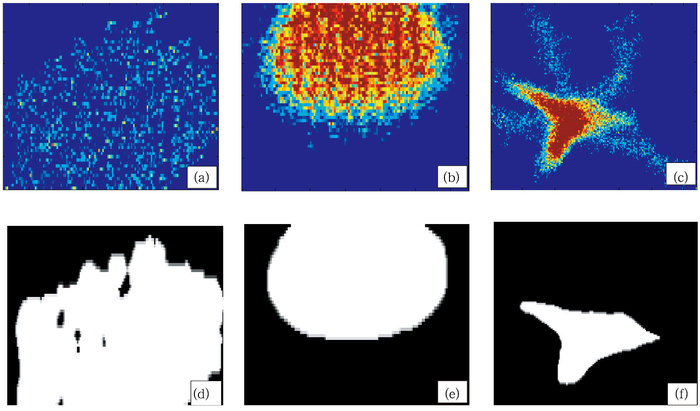图 2 PMCS所捕获异常粒子的原图(a, b, c)以及所对应的二值图(d, e, f) Fig. 2 Original images (a, b, c) and binary images (d, e, f) of outlet raindrop obtained by PMCS

 $P = \frac{{{P_r}}}{{{P_b}}},{\rm{ }}E = \sum b{w_{{\rm{edge}}}},{\rm{ }}F = \frac{{{L^2}}}{{4\pi S}}$ (1)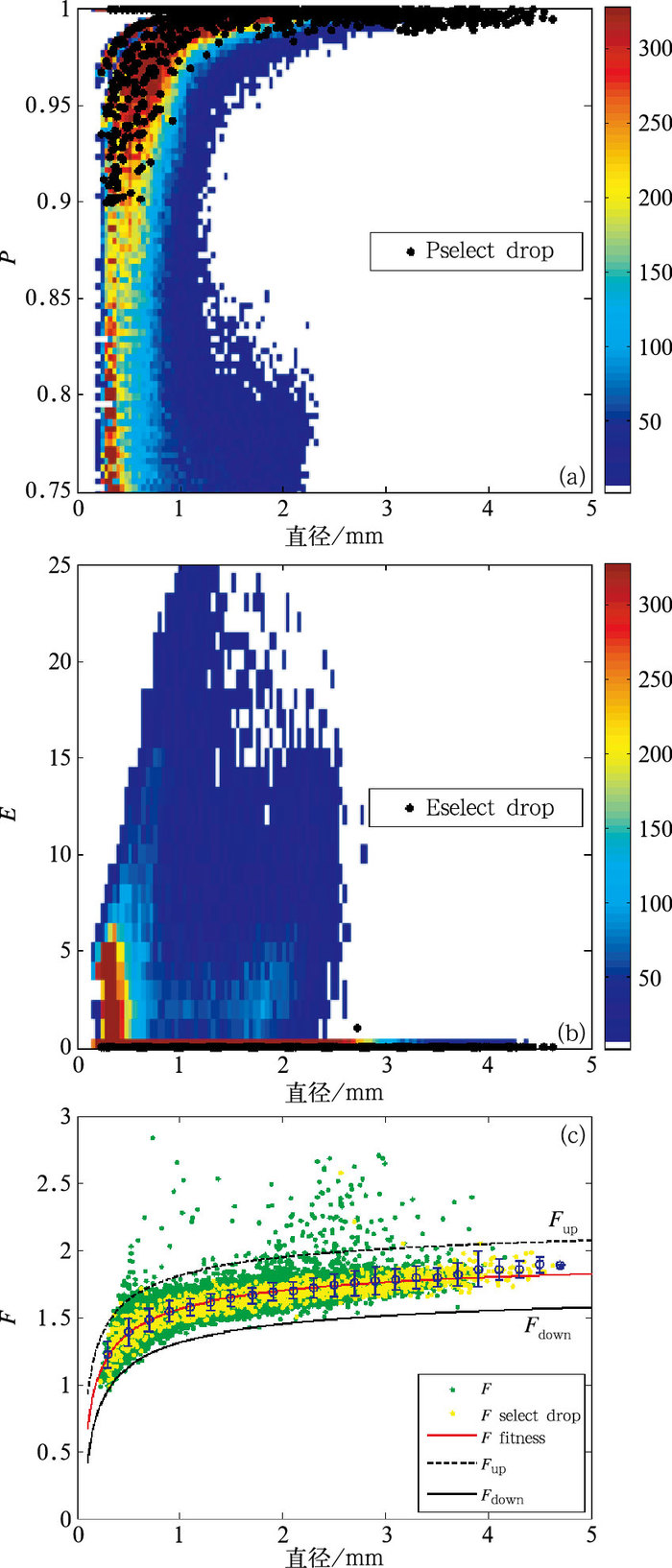图 3 有效雨滴图像的(a)空隙度P随直径的变化, (b)边缘像元数E随直径的变化, (c)形状参数F随直径变化 Fig. 3 (a) Separation versus diameter, (b) edges pixels versus diameter, (c) shape parameters versus diameter for each selected raindrop image
 $\left\{ \begin{array}{l} P \ge 0.9,{\rm{ }}E \le 1,{\rm{ }}{F_{up}} \le F \le {F_{{\rm{down}}}}\\ {F_{{\rm{up}}}} = - 0.5347{D^{ - 0.427}} + 2.0983 + 0.25\\ {F_{{\rm{down}}}} = - 0.5347{D^{ - 0.427}} + 2.0983 - 0.25 \end{array} \right.$ (2)
3 基于Akima插值的数字雨滴图像矢量化方法 3.1 雨滴图像矢量化算法设计

PMCS所捕获的雨滴图像，通过图像处理算法，最终得到是雨滴的二值图，受数值图像分辨率的限制，其边缘呈锯齿状，形如图 4中的阴影部分，而真实形状(红色实线)被掩盖在数值图像的二值化边缘图像(深灰色部分)之中，需要采用适当的矢量化方法才能提取出雨滴的真实形状。从图 4中可以看出，数值雨滴边缘图的拐点可以作为矢量化的特征点，尤其是内角点以及外角点具有与真实形状一致的变化趋势，如果分别以内外角点为基础将其连续化并取两者平均，可进一步达到矢量化雨滴数值图像的目的。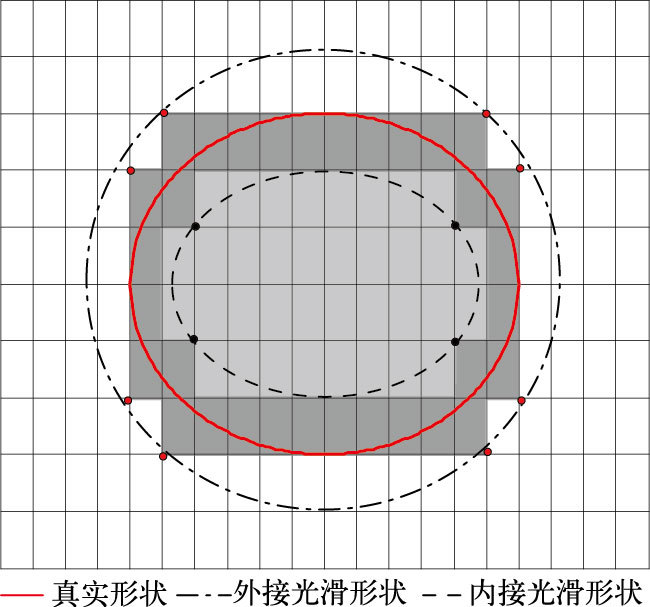图 4 数值图像与矢量形状对比 (深灰色表示真实形状对应的数值图边缘) Fig. 4 Comparison between digital and vector images (Dark grey represents the edge of digital images)

 $\begin{array}{*{20}{l}} {\quad \quad \quad \quad \rho = \sqrt {x_\rho ^2 + y_\rho ^2} }\\ {\theta = \left\{ {\begin{array}{*{20}{l}} {{\rm{arctan}}(\left| {\frac{{{y_\rho }}}{{{x_\rho }}}} \right|),{x_\rho } > 0,{y_\rho } > 0}\\ {{\rm{arctan}}(\left| {\frac{{{x_\rho }}}{{{y_\rho }}}} \right|) + \frac{\pi }{2},{x_\rho }{\rm{ < }}0,{y_\rho } > 0}\\ {{\rm{arctan}}(\left| {\frac{{{y_\rho }}}{{{x_\rho }}}} \right|) + \pi ,{x_\rho }{\rm{ < }}0,{y_\rho }{\rm{ < }}0}\\ {{\rm{arctan}}(\left| {\frac{{{x_\rho }}}{{{y_\rho }}}} \right|) + \frac{{3\pi }}{2},{x_\rho } > 0,{y_\rho }{\rm{ < }}0} \end{array}} \right.} \end{array}$ (3)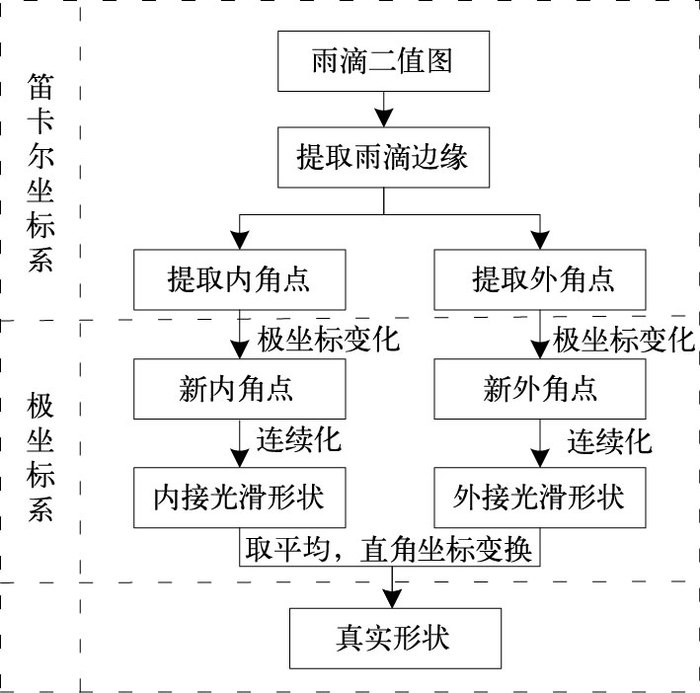图 5 实测雨滴形状处理流程 Fig. 5 Flowchart of the real raindrop dealing algorithm
3.2 角点连续化方法

 $\left\{ \begin{array}{l} x = {c_1}\sqrt {1 - {{(\frac{y}{{{c_2}}})}^2}} \left[ {{\rm{co}}{{\rm{s}}^{ - 1}}(\frac{y}{{{c_3}{c_2}}})} \right]{\rm{ }}\left[ {{c_4}{{(\frac{{{y_4}}}{{{c_2}}})}^2} + 1} \right],\\ \quad {D_{{\rm{eq}}}} \ge 1.5{\rm{ mm}}\\ {x^2} + {y^2} = D_{{\rm{eq}}}^2,{\rm{ }}{D_{eq}} < 1.5{\rm{ mm}} \end{array} \right.$ (4)

 $\left\{ \begin{array}{l} {c_1} = \frac{1}{\pi }(0.02914D_{{\rm{eq}}}^2 + 0.9263{D_{{\rm{eq}}}} + 0.07791)\\ {c_2} = - 0.01983D_{{\rm{eq}}}^2 + 0.4698{D_{{\rm{eq}}}} + 0.09538\\ {c_3} = - 0.06123D_{{\rm{eq}}}^3 + 1.3380D_{{\rm{eq}}}^2 - \\ \quad \quad 10.41{D_{{\rm{eq}}}} + 28.34\\ {c_4} = - 0.01352D_{{\rm{eq}}}^3 + 0.2014D_{{\rm{eq}}}^2 - 0.8964{D_{{\rm{eq}}}} + \\ \quad \quad 1.226{\rm{ for }}{D_{{\rm{eq}}}} > 4{\rm{ mm}}\\ {c_4} = 0{\rm{ for }}1.5{\rm{ mm}} \le {D_{eq}} \le 4{\rm{ mm}} \end{array} \right.$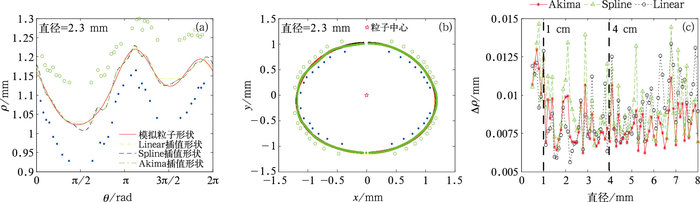图 6 模拟雨滴采用不同插值方法效果比对 (a)极坐标系下的拟合效果，(b)直角坐标系下的拟合效果，(c)拟合的平方根误差 Fig. 6 Comparison among different interpolations used in simulated raindrops (a) the fitting results in polar coordinate system, (b) the fitting results in rectangular coordinate system, (c) the mean squared error of fitting results
4 实测雨滴形状特征 4.1 雨滴平均形状特征

 $< \varepsilon > = \left| {\sum\limits_{i = 1}^n {} \frac{{{\rho _{{\rm{raindrop}},{\rm{ }}i}}}}{n} - {\rho _{{\rm{model}}}} \times } \right|\frac{1}{n}$ (5)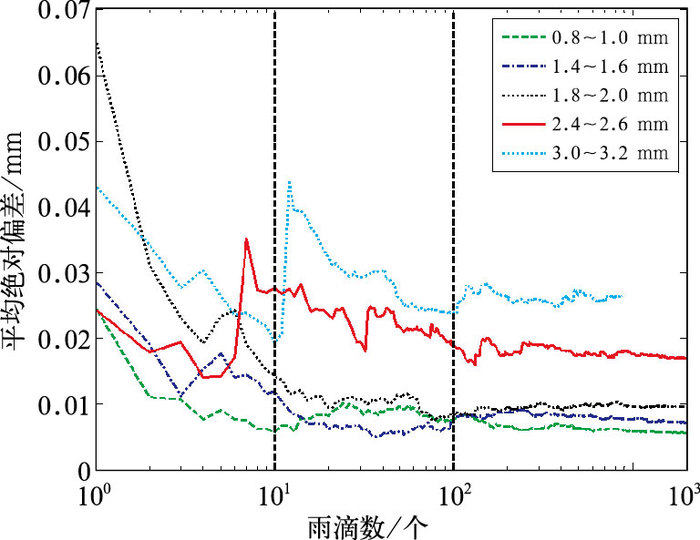图 7 不同直径范围雨滴的平均绝对偏差随雨滴个数的变化 Fig. 7 The average absolute bias versus raindrop number for different diameter range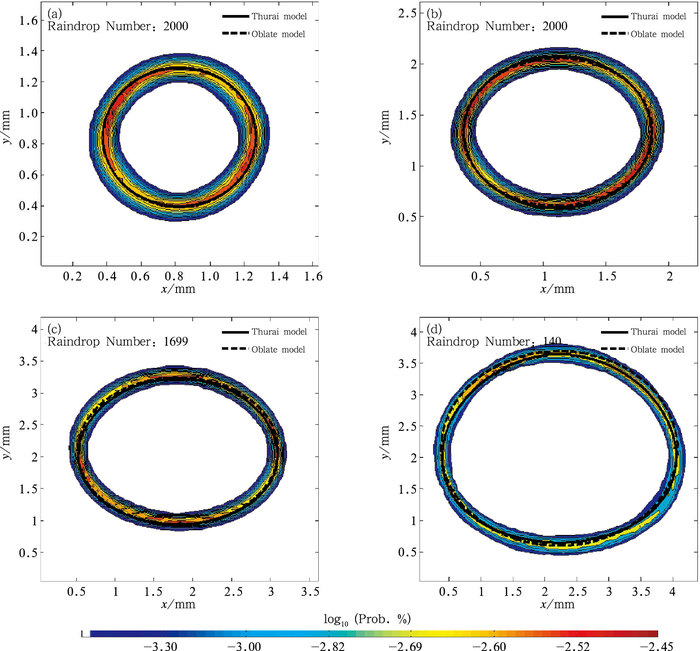图 8 不同等效直径范围雨滴统计概率(单位：%)形状 (a)0.8~1.0 mm, (b)1.4~1.6 mm, (c)2.4~2.6 mm, (d)3.4~3.6 mm Fig. 8 Drop shapes in terms of probability (unit: %) contours in different ranges of diameter (a) 0.8-1.0 mm, (b) 1.4-1.6 mm, (c) 2.4-2.6 mm, (d) 3.4-3.6 mm
4.2 轴比分布特征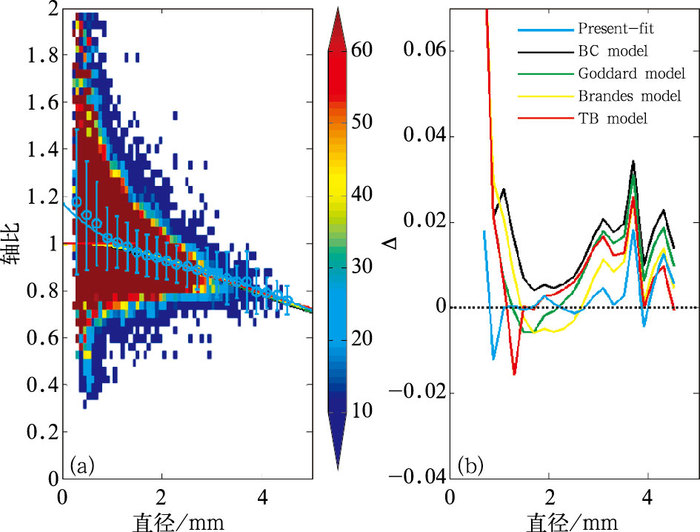图 9 实测雨滴轴比分布特征(a)和实测特征与经验分布的差异(b) Fig. 9 (a) Characteristics of real axis ratio distribution, (b) differencees in axis ratio between the mean of data and the existing models表 2 不同直径范围内实测雨滴轴比均值与标准差 Table 2 Mean and standard deviation of the axis ratio in different ranges of diameter

 $\begin{array}{l} \frac{b}{a} = 1.2508 - 0.3585{D_{{\rm{eq}}}} + 0.1598D_{{\rm{eq}}}^2 - \\ \quad \quad 0.0359D_{{\rm{eq}}}^3 + 0.0028D_{{\rm{eq}}}^4 \end{array}$ (6)

 $\frac{b}{a} = 1.0738 - 0.0683{D_{{\rm{eq}}}}$ (7)
5 结论

(1) 总体而言，雨滴形状随着等效直径的增加逐渐由圆形向椭圆形过渡，再由椭圆形向顶部凸起，底部扁平过渡。直径<1.0 mm的雨滴以圆形为主，直径在1.0~3.0 mm的雨滴以椭圆形为主，直径>3.0 mm的雨滴开始呈现较为显著的顶部凸起，底部扁平的形状。

(2) 雨滴的平均轴比随等效直径的增加而逐渐减小。其分布与经验分布基本一致，差异在±0.04范围内，当直径处于2.8~4.0 mm时，雨滴平均轴比较所有经验分布值均偏大，整体与Brandes模型更为接近，直径在3.4~3.8 mm出现峰值，表明该范围内的雨滴形状相对于经验模型具有更圆的形状特征。

(3) 基于Akima插值的数字雨滴图像矢量化方法会受雨滴二值图像测量精度的影响降低雨滴形成的提取精度，因此下一步将重点考虑雨滴图像复杂边缘变化情况，研究动态估计雨滴形状方法，降低雨滴矢量形状对二值图像精度的依赖性，进一步提高描述雨滴形状的准确性。

 梁雄贵, 2010. 基于数字化图像处理的激光打标系统的研究[D]. 成都: 电子科技大学. 廖荣伟, 张冬斌, 沈艳, 2015. 6种卫星降水产品在中国区域的精度特征评估[J]. 气象, 41(8): 970-979. DOI:10.7519/j.issn.1000-0526.2015.08.006 刘西川, 高太长, 刘磊, 等, 2013. 雨滴微物理特征研究及测量技术进展[J]. 地球科学进展, 28(11): 1217-1226. DOI:10.11867/j.issn.1001-8166.2013.11.1217 刘西川, 高太长, 刘磊, 等, 2014a. 基于粒子成像测速技术的雨滴微物理特性研究[J]. 物理学报, 63(2). DOI:10.7498/aps.63.029203 刘西川, 高太长, 刘磊, 等, 2014b. 基于粒子成像测速技术的降雪微物理特性研究[J]. 物理学报, 63(19): 199201-1. 潘旸, 宇婧婧, 廖捷, 等, 2011. 地面和卫星降水产品对台风莫拉克降水监测能力的对比分析[J]. 气象, 37(5): 564-570. DOI:10.7519/j.issn.1000-0526.2011.05.007 温龙, 刘溯, 赵坤, 等, 2015. 两次降水过程的微降雨雷达探测精度分析[J]. 气象, 41(5): 577-587. DOI:10.7519/j.issn.1000-0526.2015.05.006 张亚萍, 张勇, 廖峻, 等, 2013. 天气雷达定量降水估测不同校准方法的比较与应用[J]. 气象, 39(7): 923-929. DOI:10.7519/j.issn.1000-0526.2013.07.013 Akima H, 1970. A new method of interpolation and smooth curve fitting based on local procedures[J]. J Assoc comp Machinery, 17(4): 589-602. DOI:10.1145/321607.321609 Beard K V, Bringi V N, Thurai M, 2010. A new understanding of raindrop shape[J]. Atmos Res, 97: 396-415. DOI:10.1016/j.atmosres.2010.02.001 Beard K V, Chuang C, 1987. A new model for the equilibrium shape of raindrops[J]. J Atmos Sci, 44(11): 1509-1524. DOI:10.1175/1520-0469(1987)044<1509:ANMFTE>2.0.CO;2 Bhalwankar R, Deshpande C G, Kamra A K, 2015. Shape and oscillations of the water drops freely suspended in a horizontal electric field:a wind tunnel study[J]. J Atmos Sol-Terr Phys, 133: 169-177. DOI:10.1016/j.jastp.2015.09.004 Brandes E A, Zhang G, Vivekanandan J, 2002. Experiments in rainfall estimation with a polarimetric radar in a subtropical environment[J]. J Appl Meteor, 41(6): 674-684. DOI:10.1175/1520-0450(2002)041<0674:EIREWA>2.0.CO;2 Goddard J W F, Cherry S M, Tan J, 1994. Self-consistent measurements of differential phase and differential reflectivity in rain[D].Proceeding 1994 Geosicence and Remote Sensing Symposium. Sadena CA:IEEE. Kruger A, Krajewski W F, 2002. Two-dimensional video disdrometer:a description[J]. J Atmos Ocea Tech, (19): 602-917. Liu X C, Gao T C, Liu L, 2014. A video precipitaion sensor for imaging and velocimetry of hydrometeors[J]. Atmos Meas Tech, 7: 2037-2046. DOI:10.5194/amt-7-2037-2014 Marzuki, Randeu W L, Kozu T, et al, 2013. Raindrop axis ratios, fall velocities and size distribution over Sumatra from 2D-Video disdrometer measurement[J]. Atmos Res, 119: 23-37. DOI:10.1016/j.atmosres.2011.08.006 Michaelides S, 2008. Precipitation:Advances in Measurement, Estimation, and Prediction[M]. Berlin: Springer, 23-103. Pruppacher H R, Klett J D, 1997. Microphysics of Clouds and Precipitation (Second Revised and Enlarged Edition with An Introduction to Cloud Chemistry and Cloud Electricity)[M]. New York: Kluwer Academic Publishers, 361-444. Pruppacher H R, Pitter R L, 1971. A semi-empirical determination of shape of cloud and raindrops[J]. J Atmos Sci, 28(1): 86-94. DOI:10.1175/1520-0469(1971)028<0086:ASEDOT>2.0.CO;2 Shu Xiaojian, Gao Taichang, Liu Xichuan, et al, 2015. Research on the calibration method of precipitation micro-physical characteristics sensor[J]. Instrmentation, 2(3): 55-64. Thurai M, Bringi V N, 2005. Drop axis ratios from a 2D video disdrometer[J]. J Atmos Ocean Tech, 22: 966-978. DOI:10.1175/JTECH1767.1 Thurai M, Bringi V N, 2007. Drop axis ratios from a 2D video disdrometer[J]. Adv Geosci, 10: 85-90. DOI:10.5194/adgeo-10-85-2007 Thurai M, Huang G J, Bringi V N, et al, 2007. Drop shapes, model comparisons, and calculations of polarimetric radar parameters in rain[J]. J Atmos Ocean Tech, 24(6): 1019-1032. DOI:10.1175/JTECH2051.1 Thurai M, Bringi V N, Petersen, et al, 2010. Drop axis ratio distributions in stratiform and convective rain[D].The Sixth European Conference on Radar in Meteorology and Hydrology.